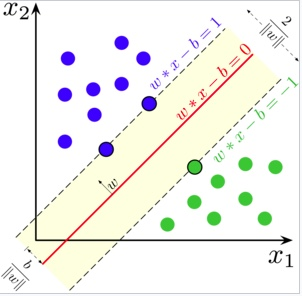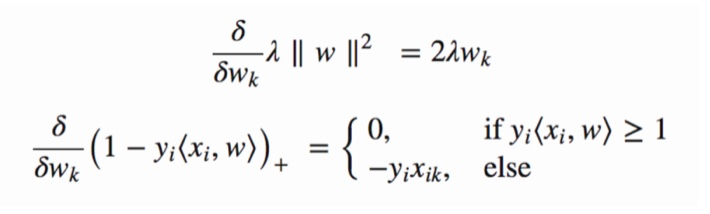# How To Use SVM In Python?

## What is SVM?

Intuitively, a good separation is achieved by the hyperplane that has the largest distance to the nearest training-data point of any class (so-called functional margin), since in general the larger the margin the lower the generalization error of the classifierSVM

### Soft-margin

$\left[ \frac { 1 } { n } \sum _ { i = 1 } ^ { n } \max \left( 0,1 - y _ { i } \left( \vec { w } \cdot \vec { x } _ { i } - b \right) \right) \right] + \lambda \| \vec { w } \| ^ { 2 }$SVM_principle

$w = w - \alpha \cdot ( 2 \lambda w )$ and $w = w + \alpha \cdot \left( y _ { i } \cdot x _ { i } - 2 \lambda w \right)$ when not correct

import pandas as pd
import matplotlib.pyplot as plt

from sklearn.utils import shuffle
from sklearn.model_selection import train_test_split
import numpy as np

df = pd.read_csv('iris.csv',index_col = False, delimiter = ',')
df = df.drop(df.index[list(range(100,150))])  # to binary classification
df = df.drop(['SepalWidth','PetalWidth'],axis= 1)
df['Species'] = np.where(df['Species']==0, -1, 1)
Y = df['Species']
X = df.drop(['Species'],axis=1)
X, Y = shuffle(X,Y)
x_train, x_test, y_train, y_test = train_test_split(X, Y, train_size=0.8)
x_train = np.array(x_train)
y_train = np.array(y_train)
x_test = np.array(x_test)
y_test = np.array(y_test)

y_train = y_train.reshape(len(x_train),1)
y_test = y_test.reshape(len(x_test),1)

train_f1 = x_train[:,0]
train_f2 = x_train[:,1]

train_f1 = train_f1.reshape(len(x_train),1)
train_f2 = train_f2.reshape(len(x_train),1)

w1 = np.zeros((len(x_train),1))
w2 = np.zeros((len(x_train),1))

epochs = 1
alpha = 0.0001
W = np.zeros((len(x_train),2))
while(epochs < 10000):
y = w1 * train_f1 + w2 * train_f2
prod = y * y_train
print(epochs)
count = 0
for val in prod:
if(val >= 1):
cost = 0
w1 = w1 - alpha * (2 * 1/epochs * w1)
w2 = w2 - alpha * (2 * 1/epochs * w2)

else:
cost = 1 - val
w1 = w1 + alpha * (train_f1[count] * y_train[count] - 2 * 1/epochs * w1)
w2 = w2 + alpha * (train_f2[count] * y_train[count] - 2 * 1/epochs * w2)
count += 1
epochs += 1

from sklearn.metrics import accuracy_score

## Clip the weights
index = list(range(len(x_test),len(x_train)))
w1 = np.delete(w1,index)
w2 = np.delete(w2,index)

w1 = w1.reshape(len(x_test),1)
w2 = w2.reshape(len(x_test),1)
## Extract the test data features
test_f1 = x_test[:,0]
test_f2 = x_test[:,1]

test_f1 = test_f1.reshape(len(x_test),1)
test_f2 = test_f2.reshape(len(x_test),1)
## Predict
y_pred = w1 * test_f1 + w2 * test_f2
predictions = []
for val in y_pred:
if(val > 1):
predictions.append(1)
else:
predictions.append(-1)

print(accuracy_score(y_test,predictions))

## Output


or

from sklearn.svm import SVC
from sklearn.metrics import accuracy_score

clf = SVC(kernel='linear')
clf.fit(x_train,y_train)
y_pred = clf.predict(x_test)
print(accuracy_score(y_test,y_pred))


Welcome to share or comment on this post: